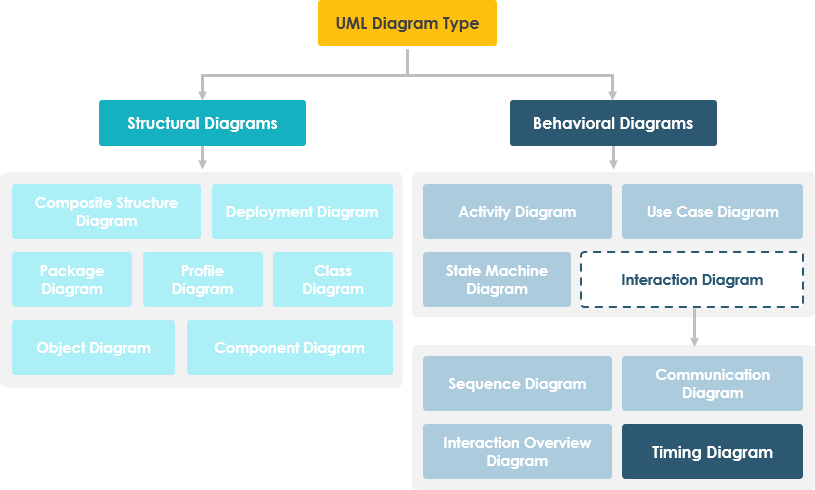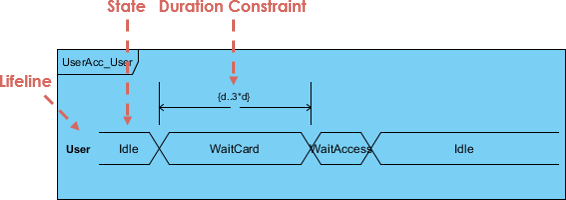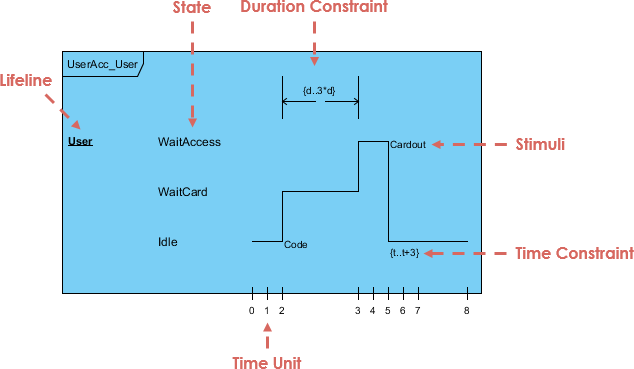1. Home
2. Docs
3. Chapter 5. UML & Requirement Diagram
4. 12. Timing Diagram

# 12. Timing Diagram

Timing diagrams are used to explore the behaviors of objects throughout a given period of time. You can use this diagram to provide a snapshot of timing data for a particular part of a system. A timing diagram is a special form of a sequence diagram. The differences between timing diagram and sequence diagram are the axes are reversed so that the time is increased from left to right and the lifelines are shown in separate compartments arranged vertically.

A timing diagram in the Unified Modeling Language 2.0 is a specific type of interaction diagram, where the focus is on timing constraints.### Concise notation VS Robust notation

There are two basic flavors of timing diagram: the concise notation, and the robust notation.

Concise NotationRobust notation